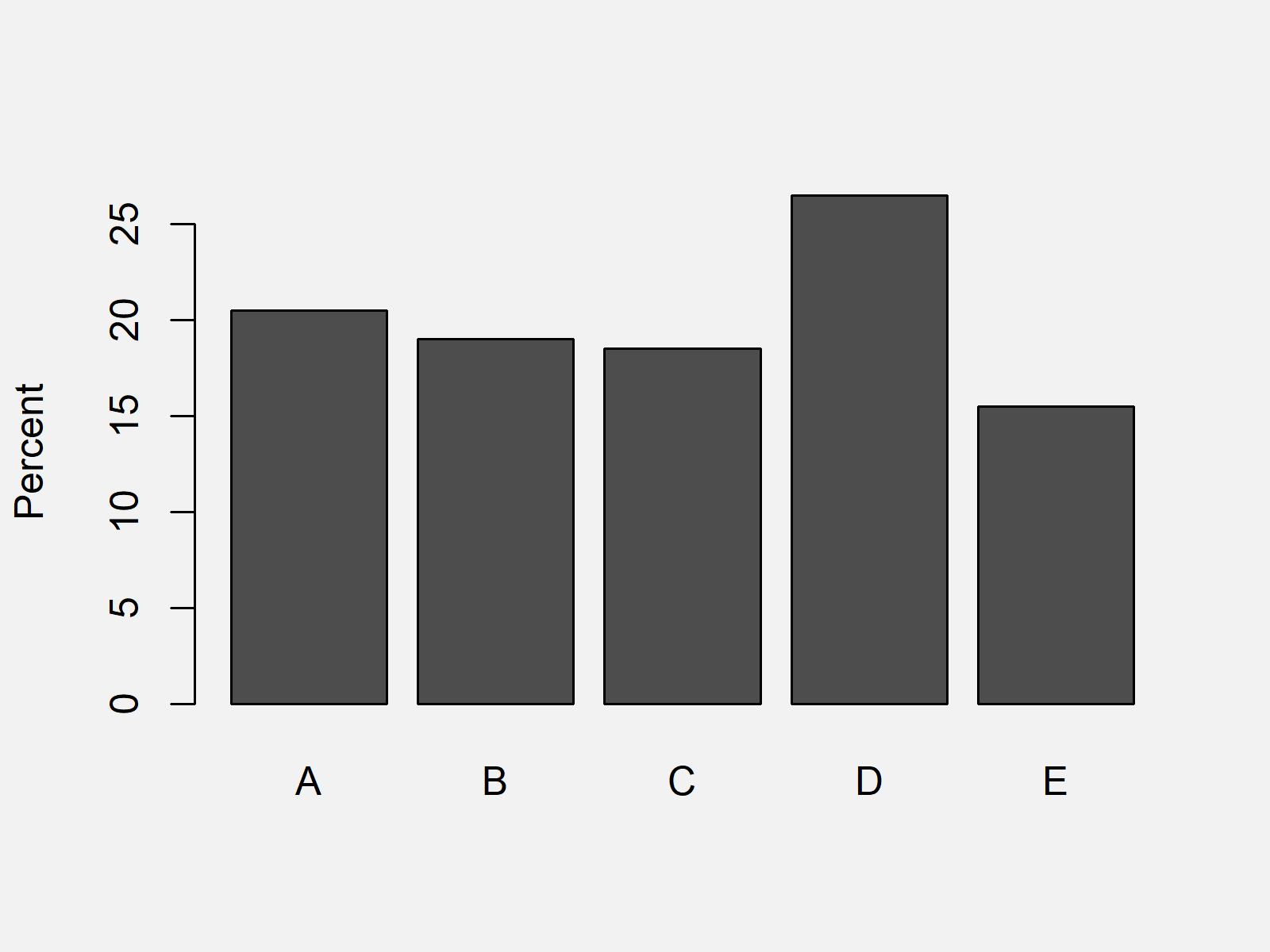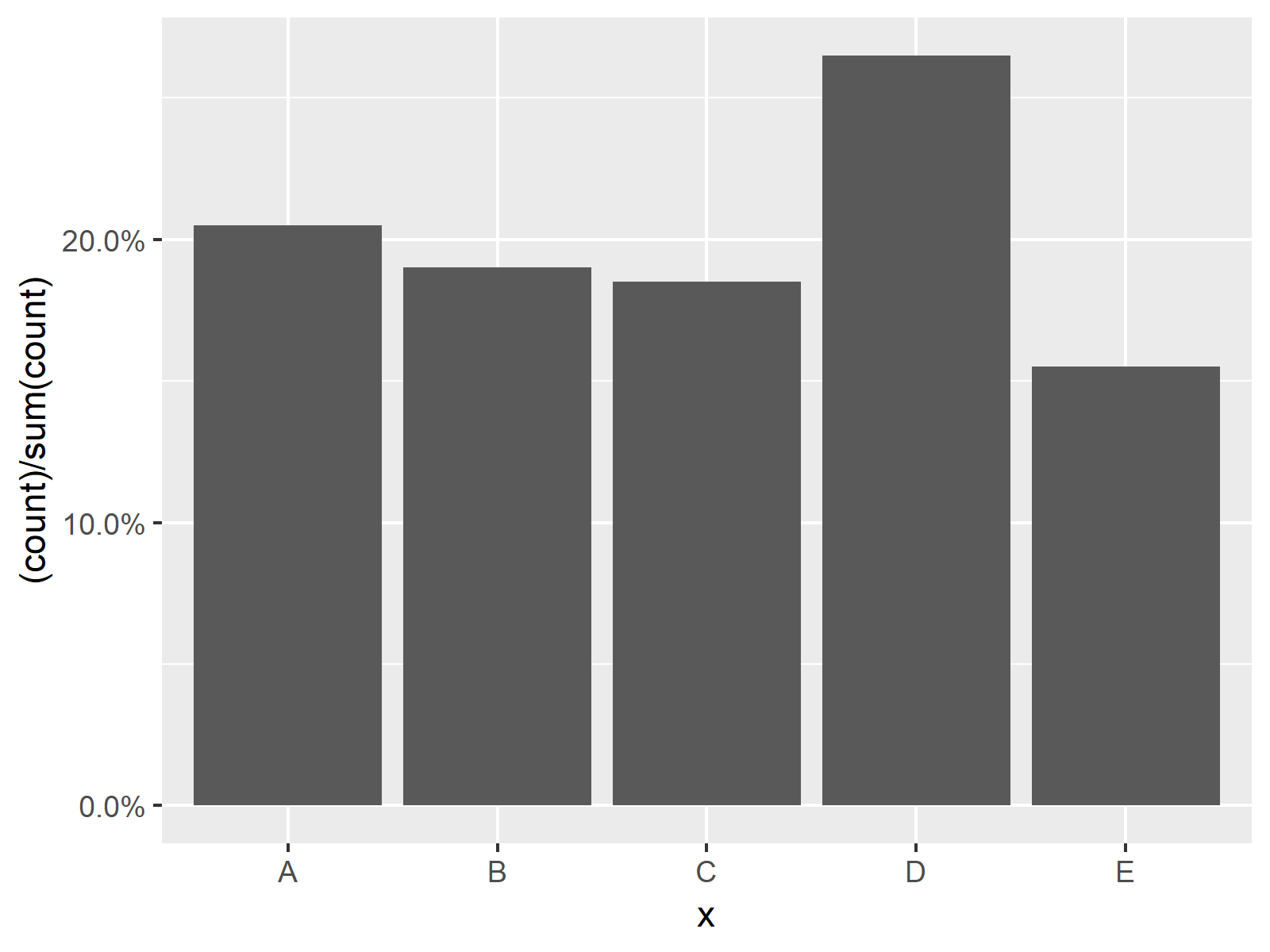# Plotting Categorical Variable with Percentage Points Instead of Counts on Y-Axis in R (2 Examples)

In this R article you’ll learn how to draw categories of a variable with percentage points on the y-axis.

The post consists of two examples for the plotting of data in R. To be more precise, the page looks as follows:

Here’s the step-by-step process!

## Creating Example Data

We’ll use the data below as basement for this R programming tutorial:

```set.seed(927456)                                   # Creating example data
data <- data.frame(x = sample(LETTERS[1:5],
200,
replace = TRUE))
#   x
# 1 A
# 2 C
# 3 D
# 4 C
# 5 E
# 6 C```

As you can see based on the previous output of the RStudio console, our example data has only one column consisting of categorical values.

## Example 1: Barchart with Percentage on Y-Axis Using barplot() Function of Base R

The following R programming code explains how to show percentage points of the y-axis of a barplot created with the basic installation of the R programming language.

For this task, we first have to convert our data frame to a matrix consisting of the percentages of each category:

`data_perc <- t(prop.table(table(data\$x))) * 100    # Convert data to probability table`

Now, we can draw our barchart with percentage points on the y-axis as shown below:

`barplot(data_perc, ylab = "Percent")               # Draw barchart with Base R`As shown in Figure 1, the previous code created a Base R barchart with %-points on the y-axis.

## Example 2: Barchart with Percentage on Y-Axis Using ggplot2 Package

This section illustrates how to plot a ggplot2 graph with percentage points instead of counts on the y-axis.

We first need to install and load the ggplot2 package:

```install.packages("ggplot2")                        # Install & load ggplot2 package
library("ggplot2")```

Furthermore, we have to install and load the scales package:

```install.packages("scales")                         # Install & load scales package
library("scales")```

Now, we can draw our graphic as follows:

```ggplot(data, aes(x)) +                             # Draw barchart with ggplot2 package
geom_bar(aes(y = (..count..)/sum(..count..))) +
scale_y_continuous(labels = percent)```The output of the previous R programming code is shown in Figure 2 – A ggplot2 barplot with % on the y-axis instead on counting numbers.

## Video, Further Resources & Summary

In case you need further explanations on the examples of this tutorial, you could have a look at the following video of my YouTube channel. In the video, I explain the R programming codes of the present article:

Please accept YouTube cookies to play this video. By accepting you will be accessing content from YouTube, a service provided by an external third party.If you accept this notice, your choice will be saved and the page will refresh.

Furthermore, you could have a look at the other articles of this homepage: